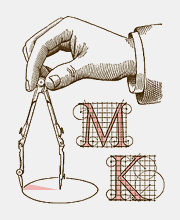Rhombi 7

Inscribe a rhombus into the given rectangle so that two of its vertices are the points A and B and the other two lie on the perimeter of the rectangle. To draw the rhombus, use the Polygon tool.

The better of two solutions is the one with the smaller number of new lines. If two solutions have equally many new lines, the numbers of new points are compared.